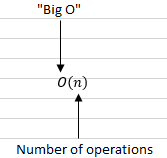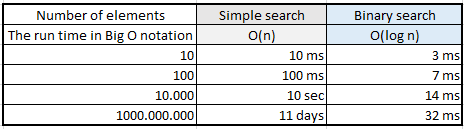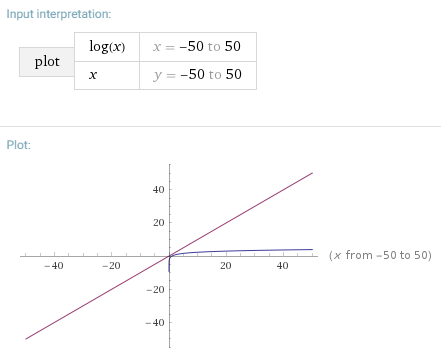## Big O Notation

As a computer scientist, if you are working on an important piece of software, you will likely need to be able to estimate how fast some algorithm or other going to run.

Big O notation is used in computer science to describe the performance or complexity of an algorithm. Actually Big O notation is special symbol that tells you how fast an algorithm is. Of course you’ll use predefined algorithms often — and when you do, it’s vital to understand how fast or slow they are.

#### What Big O notation looks like?This tells you the number of operations an algorithm will make. It’s called Big O notation because you put a “Big O” in front of the number of operations.

#### Big O establishes a worst-case run time

Say you are a doctor who is treating Harry Abbit, you might look into the electronic records that are related to Harry Abbit’s medical history (he is the first person in a list). Lets consider the situation when his life depends on all available medical data. Suppose you’re using simple search to look for a person in the electronic records. You know that simple search takes O(n) time to run, so you’ll have to look through every single entry for Abbit. Of course, you’ve noticed that Abbit is the first entry, so you didn’t have to look at every entry — you found it on the first try.

Did this algorithm take O(n) time? Or did it take O(1) time because you found the person on the first try?

In this case, that’s the best-case scenario. But Big O notation is about the worst-case scenario. That’s O(n) time (simple search still takes). It’s a reassurance that simple search will never be slower than O(n) time.

#### Algorithm running times grow at different rates

Let’s assume it takes 1 millisecond to check one entry. With simple search, the doctor has to check 10 entries, so the search takes 10 ms to run. On the other hand, he only has to check 3 elements with binary search algorithm (log10 is roughly 3), so that search takes 3 ms to run.

But realistically, the list will have more than a hundred elements.

If it does, how long will simple search take? How long will binary search take?

The run time for simple search with 1 billion items will be 1 billion ms, which is 11 days. The problem is, the run times for binary search and simple search don’t grow at the same rate.So as the list of numbers gets bigger, binary search becomes a lot faster than simple search. That is, as the number of items increases, binary search takes a little more time to run. But simple search takes a lot more time to run. So as the list of numbers gets bigger, binary search becomes a lot faster than simple search.

That’s why it’s not enough to know how long an algorithm takes to run — you need to know how the running time increases as the list size increases. That’s where Big O notation comes in.

#### Big O notation lets you compare the number of operations

For example, suppose you have a list of size n. Simple search needs to check each element, so it will take n operations. The run time in Big O notation is O(n).

Where are the seconds?

There are none — Big O doesn’t tell you the speed in seconds. Big O notation lets you compare the number of operations. It tells you how fast the algorithm grows.Most common running times for algorithms

A list of the most common running times for algorithms in terms of Big O notation.  Here are five Big O run times that you’ll encounter a lot, sorted from fastest to slowest:

1. O(log n), also known as log time.  Example: Binary search.
2. O(n), also known as linear time.  Example: Simple search.
3. O(n * log n) Example: A fast sorting algorithm, like quicksort (coming up in chapter 4).
4. O(n2) Example: A slow sorting algorithm, like selection sort (coming up in chapter 2).
5. O(n!) Example: A really slow algorithm, like the traveling salesperson (coming up next!).

This article only covers the very basics of Big O. For a more in-depth explanation take a look at their respective FreeCodeCamp guides for algorithms.